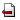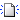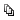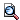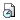In the first part, I present a modified Allen-Cahn equation that can be used for image processing tasks. I will summarize the previous developments in active contours models that also provide a motivation for the phase field approach. A finite difference approximation will be presented together with a number of numerical results showing the function of the algorithm.
The second part of my talk concerns numerical solution of the Cahn-Hilliard equation, a well-known fourth-order nonlinear PDE model of phase separation in binary mixtures. I will show its several non-degenerate and degenerate forms, mention its relation to the motion of curves and study the convergence of a finite difference scheme. To conclude this part of the talk, I show again some numerical results.
The third, last part of the talk will deal with a two-scale model of liquid phase epitaxy. First, a simpler model will be presented together with a numerical scheme and some computational results. Then, the full two-scale model will be shown with a preliminary approach to its numerical solution.

ご不明な点がございましたら、事務局: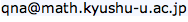までお問い合わせ下さい。